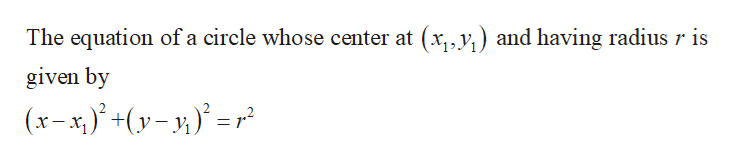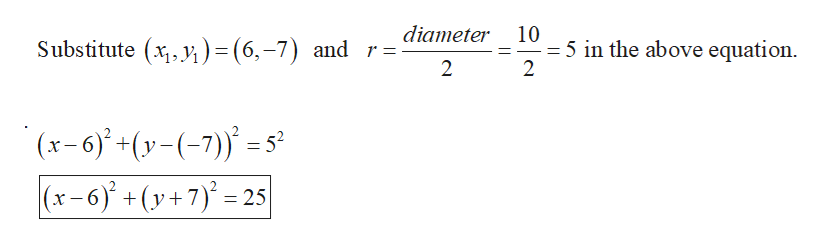# How do I find the standard form equation for a circle with center point at (6,-7) and diameter of 10?

Question
4 views

How do I find the standard form equation for a circle with center point at (6,-7) and diameter of 10?

check_circle

Step 1help_outlineImage TranscriptioncloseThe equation of a circle whose center at (x,, y,) and having radius r is given by (x- x;)° +(y- y,)° = r? fullscreen
Step 2help_outlineImage Transcriptionclosediameter 10 = 5 in the above equation. 2 Substitute (x,y, ) = (6,-7) and r= 2 (x-6)* +(y-(-7) = 53? |(x-6)' +(y+7)' = 25 fullscreen

### Want to see the full answer?

See Solution

#### Want to see this answer and more?

Solutions are written by subject experts who are available 24/7. Questions are typically answered within 1 hour.*

See Solution
*Response times may vary by subject and question.
Tagged in

### Math